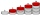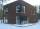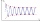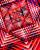# Arithmetic progression + natural numbers - math problems

#### Number of problems found: 32

• AM of three numbersThe number 2010 can be written as the sum of 3 consecutive natural numbers. Determine the arithmetic mean of these numbers.
• AP membersWhat is the value of x2, x3, x4, x5…of the terms (of arithmetic progression) when x1 = 8 and x6 = 20?
• Negative differenceFind four arithmetic progression members between 7 and -6.
• Real estateThe residential house has three entrances numbered by odd numbers arithmetic progression. The sum of the two numbers on the corner entrances is 50. Calculate the highest of these three numbers.
• Progression-12, 60, -300,1500 need next 2 numbers of pattern
• Sum of four numbersThe sum of four consecutive natural numbers is 114. Find them.
• Find the sumFind the sum of all natural numbers from 1 and 100, which are divisible by 2 or 5
• Sum of the seventeen numbersThe sum of the 17 different natural numbers is 154. Determine the sum of the two largest ones.
• Sum 1-6Find the sum of the geometric progression 3, 15, 75,… to six terms.
• 10th termWhat is the 10th term of the Arithmetic Progression if x1=4 and d=5?
• Finite arithmetic sequenceHow many numbers should be inserted between the numbers 1 and 25 so that all numbers create a finite arithmetic sequence and that the sum of all members of this group is 117?
• What areWhat are the four terms between 4 and 19?
• Simple sequenceContinue with this series of numbers: 1792,448, 112, _, _
• The sumThe sum of the first 10 members of the arithmetic sequence is 120. What will be the sum if the difference is reduced by 2?
• Sum of odd numbersFind the sum of all odd integers from 13 to 781.
• SequenceIn the arithmetic sequence is given: Sn=2304, d=2, an=95 Calculate a1 and n.
• Odd numbersThe sum of four consecutive odd numbers is 1048. Find those numbers ...
• NumbersDetermine the number of all positive integers less than 4183444 if each is divisible by 29, 7, 17. What is its sum?
• Find the meanFind the number between 13 and 29.
• CalculateCalculate the sum of all three-digit natural numbers divisible by five.

Do you have an interesting mathematical word problem that you can't solve it? Submit a math problem, and we can try to solve it.

We will send a solution to your e-mail address. Solved examples are also published here. Please enter the e-mail correctly and check whether you don't have a full mailbox.

Please do not submit problems from current active competitions such as Mathematical Olympiad, correspondence seminars etc...

Arithmetic progression - math problems. Natural numbers - math problems.